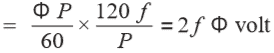# EMF Equation of an Alternator and Synchronous Generator

## EMF Equation of an Alternator  and AC Generator

An alternator or AC generator (also known as synchronous generator or dynamo) is a device which converts mechanical energy into electrical energy.

When we supply the magnetizing current by DC shunt generator through two slip rings (in recent alternators, they use electronic starting system instead of slip rings and commutators) because the field magnets are rotating. keep in mind that most alternators use a rotating magnetic field with a stationary armature.

When the rotor rotates, the stator conductors which are static in case of alternator cut by magnetic flux , they have induced EMF produced in them (according to Faraday’s law of electromagnetic induction which states that if a conductor or coil links with any changing flux, there must be an induced EMF in it.

Note: We will discuss the construction, working & operation, types of Alternators in details in our next posts.This induced EMF can be found by the EMF equation of the alternator which as follow:

Lets,

P = No. of poles

Z = No. of conductors or Coil sides in series/phase i.e. Z = 2T…Where T is the number of coils or turns per phase (Note that one turn or coil has two ends or sides)

f = frequency of induced EMF in Hz

Φ = Flux per pole (Weber)

N = rotor speed (RPM)

Kc or KP = Cos α/2

If induced EMF is assumed sinusoidal then,

K = Form factor = 1.11

In one revolution of the rotor i.e. in 60/N seconds, each conductor is cut by a flux of ΦP Webers.

dΦ = ΦP and also dΦ = 60/N seconds

But we know that:

f = PN / 120 or N= 120f / P

Putting the value of N in Equation (i), we get,

Average value of EMF per conductor =(N= 120f/P)

If there are Z conductors in series per phase,

then synchronous generator average E.M.F per phase = 2 f Φ Z Volts = 4 f ΦT Volts ….. (Z = 2T)

Also we know that;

Form Factor = RMS Value / Average Value

= RMS value = Form Factor x Average Value,

VAV = 1.11 x 4fΦT = 4.44fΦT Volts.

And the actual available voltage of generator per phase

VPH = 4.44 KC KD f ΦTPH

V = 4.44 Kf KC KD f ΦT Volts.

Where:

• V = Actual generated Voltage per phase
• KC = Coil Span Factor or Pitch Factor
• KD = Distribution Factor
• Kf = Form Factor
• f = frequency
• T = Number of coils or number of turns per phase

Note: If alternator or AC generator is star connected as usually the case, then the Line Voltage is √3 times the phase voltage as derived from the above equation.

Related Posts:

### Electrical Technology

Excellent Post About Emergency MELTDOWN Generators Shutdown and <a href="http://www.adpower.ae/&quot; rel="nofollow">Ac generator parts</a>.<br />

2. Tyagi says:

Its very usefull……thanxxx

3. satyajeet kakati says:

Nice but little lendi

4. Vijith says:

Generator kV to hp Calculation explain …

5. Arinda Clever says:

what causes the breakdown of electricity